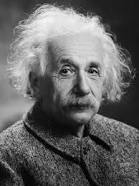## Thursday, November 12, 2015

### Einstein’s mistake

When Einstein formulated in 1915 his theory of general relativity, he soon applied it to the entire universe, deriving the following cosmological equation:

It is curious that this equation is identical to the equation that would result from Newton’s theory of gravitation. There is only one difference: constant k represents, in Newton’s case, the total energy of the universe; in Einstein’s case, its curvature.
Each term of this equation contains a universal constant. Besides k, G is the gravitational constant; L is called the cosmological constant, whose interpretation is not clear. Einstein initially thought he could eliminate this term by making L = 0, which simplifies the equation and makes it analytically solvable. Then he discovered that the solution, in that case, was a universe in constant expansion. Since he believed that the universe had to be stationary, he decided to assign the constant a critical value L = Lc, to make it be so.
Unfortunately for Einstein, a few years later two things were discovered:
• The universe is actually expanding (Lemaître and Hubble).
• Einstein’s universe with L=Lc is stationary, but unstable. Any small disturbance would make it go into an endless expansion or a contraction to zero volume.Albert Einstein
When he realized these two things, Einstein said that introducing the cosmological constant was the biggest mistake he had made. However, in 1998, when the accelerating expansion of the universe was discovered , cosmologists recovered the cosmological constant to explain this phenomenon, and equated the third term of Einstein’s equation with the mysterious dark energy.
The problem is we do not know what dark energy may be. If it exists (this is not yet proven) it would probably be a new fundamental interaction of the universe, apart from the four we know. This would be as big news as the discovery of radioactivity in the late nineteenth century: a whole new field would be open to physics. Also its value seems to be quite small, very close to zero, which does not square with quantum field theories that have tried to explain it as the vacuum energy, according to which its value should be 10120 higher. M.P. Hobson and his colleagues have called this the worst theoretical prediction of the history of physics.
In conclusion, we have two possibilities:

• If dark energy does not exist, if the accelerating universe were an artifact or could be explained otherwise (some physicists are analyzing these two possibilities), perhaps Einstein was right when he said that introducing the cosmological constant was the biggest mistake he had made.
• But if dark energy really exists and the cosmological constant has the value the standard model assigns to it (10-52 m-2), then Einstein’s mistake would have been saying the words quoted in the previous paragraph.
The same post in Spanish
Thematic thread on Standard Cosmology: Preceding Next
Manuel Alfonseca